Lee Plath

•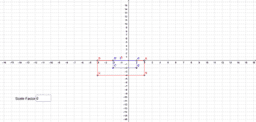Find the scale factor

Activity

Lee Plath

•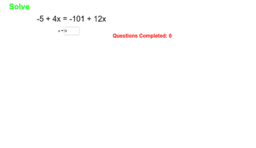Solve equations with variables on both sides.

Activity

Lee Plath

•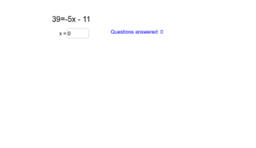Solving two-step equations.

Activity

Lee Plath

•Explore reflections

Activity

Lee Plath

•Parallel lines cut by a transversal.

Activity

Lee Plath

•Parallel lines cut by a transversal

Activity

Lee Plath

•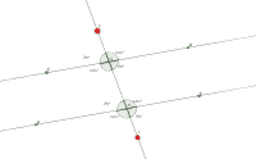Parallel lines cut by a transversal

Activity

Lee Plath

•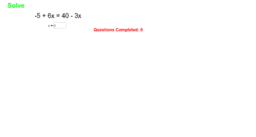Solve the equations

Activity

Lee Plath

•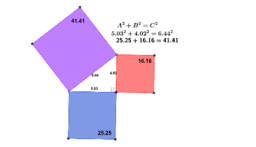Pythagorean theorem

Activity

Lee Plath

•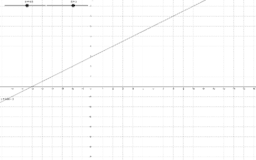Slope intercept

Activity

Lee Plath

•Algebraic dilations

Activity

Lee Plath

•algebra dilations

Activity

Lee Plath

•Find the scale factor of the dilation

Activity

Lee Plath

•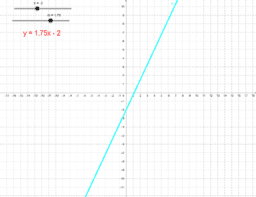Slope intecept form.

Activity

Lee Plath

•Adjusting the scale factor of a dilation

Activity

Lee Plath

•Algebra dilations

Activity

Lee Plath

•Finding area and perimeter

Activity

Lee Plath

•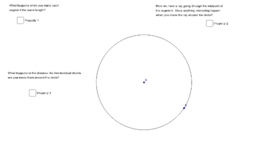Exploring Chords

Activity

Lee Plath

•Midpoints of triangles

Activity

Lee Plath

•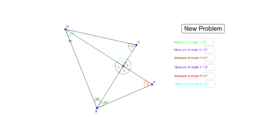Activity

Lee Plath